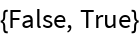#Function Repository Resource:

# Terse

An operator form of Short

Contributed by: Seth J. Chandler
 ResourceFunction["Terse"][n] gives an operator form of Short that will shorten the expression it is passed to approximately n lines.

## Examples

### Basic Examples

Output a short form of 100,000 integers in 5 lines:

 In:=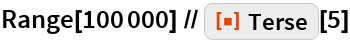Out=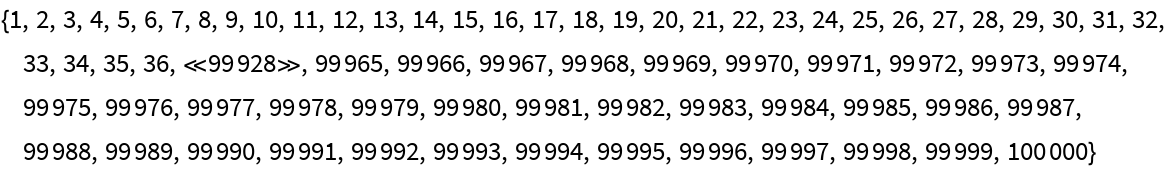Output a short form of 100,000 integers, usually in a line or two:

 In:=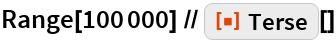Out=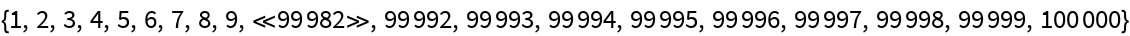### Scope

A prefix form of the Terse operator:

 In:=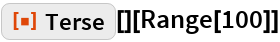Out=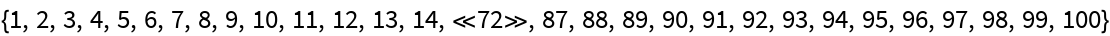An infix form of the Terse operator:

 In:=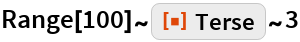Out=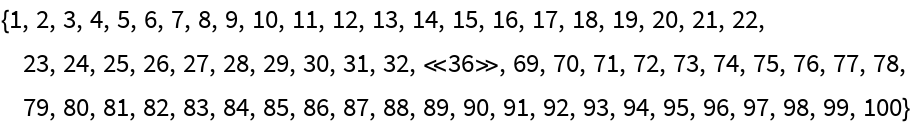### Applications

Using Terse in composition with other operators can shorten output, as shown in the following comparison:

 In:=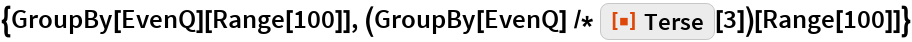Out=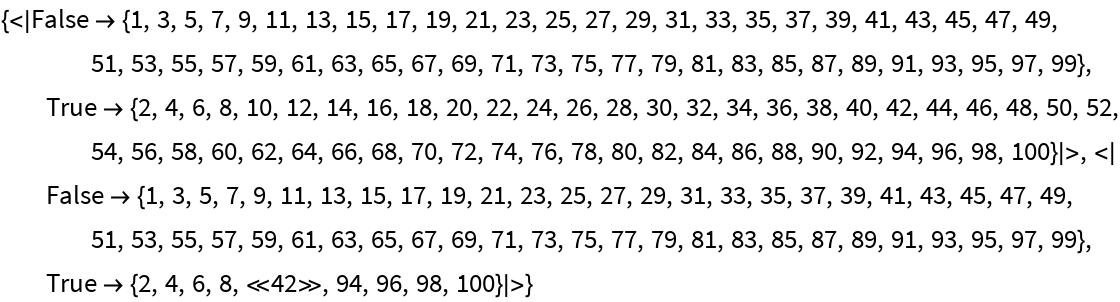### Properties and Relations

An idea behind Terse, illustrated by a comparison of the expressions in the following list, is that it is less distracting than starting code with Short or a postfix form of something like Short[#,5]&:

 In:=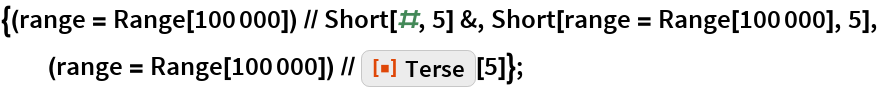### Possible Issues

Terse is like Short in that it requires parentheses surrounding the assignment in the following code if the intent is to let the variable range hold Range rather than its Short form:

 In:=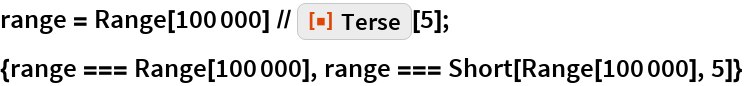Out=